Mobile QR Code1. (Department of the Electronics and Robotics Engineering, Busan University of Foreign Studies/ Busan, Korea teju.thalluri@gmail.com, {htchung, kyoojae}@bufs.ac.kr )

Electrical vehicle, Battery characteristics, State of charge, Depth of discharge, Machine learning algorithms and kNN method

1. Introduction

High environmental pollution and energy crises make natural and high energy storage devices very much necessary in this modern era . Out of all high-energy storage devices, lithium batteries have gained much attention due to their long cycle life and ecofriendly characteristics . These batteries are extensively used in transportation, electric vehicles, bicycles, vacuum cleaners, and smart phones [3-5].

Lithium batteries have excellent heat resistance when compared to lead batteries, but it was found that a battery can be damaged and also cause explosion . Hence, accurate measurements and SOC estimation are very much required to improve the efficiency and ensure safety when using the battery . The SOC is the important parameter in a Battery Management System (BMS).

Many researchers have focused their research on the safety of lithium batteries with SOC estimation before complete degradation. However, it is not easy to estimate SOC accurately due to limited computational power . The research on the comparison of different types of batteries is very limited.

Many methods have been implemented for SOC estimation. Mostly, they use battery cell estimations, but comparisons between two different batteries for SOC estimation are scarce. Estimating the SOC of a battery results in high efficiency and safety of the battery .

In this study, the SOC estimation of cells in lithium battery packs using kNN machine learning is proposed. The kNN algorithm is one of the most used machine learning algorithms due to its advantages compared to other methods, such as linear regression, support vector machine, etc. The main advantages with kNN are: 1) kNN makes predictions very quickly by calculating similarity between the input samples in each training step, 2) it requires low calculation time, and 3) it is easy to interpret the output.

The relation between voltage and SOC is evaluated after charging and discharging battery cells at room temperatures. The SOC of a battery pack is estimated by learning with collected sensor data. In this study, SOC estimation was done and compared with experimental and kNN methods. The error difference was calculated and compared during a charging and discharging process.

In section 2, we explain about the SOC estimation process in an electrochemical and equivalent circuit model. In section 3, we explain about training of the kNN model to predict SOC. Section 4 is about the experiment process, and results are explained.

2. Analysis of Battery Model for Charac- teristic Evaluation

The battery characteristics such as voltage, current, and temperature are the main parameters to be monitored to ensure proper functioning and safety of the battery before degradation. In this study, these parameters were evaluated to understand the behavior of each battery with an electrochemical and equivalent circuit model.

2.1 Battery SOC Estimation with Electro-Chemical Model

The battery characteristics can be measured with a physical model and also with equivalent circuit models. In a physical model, the lithium-ion battery mainly consists of three domains: a negative electrode, separator, and positive electrode. The structure of a battery and corresponding governing equations of the physical modeling are shown in Fig. 1.

The major physical equations describing the physics of a battery are described below as x-axis staring from negative to positive current collectors, r-axis along the radius direction of a solid electrode particle. The transportation of Li ions in the solid phase is modeled by diffusion equations that are shown in Eqs. (1) and (2). These represent the lithium-ion transportation in electrolyte .

(1)
$\frac{\partial C_{s}\left(x,r,t\right)}{\partial t}=\frac{1}{r^{2}}\frac{\partial }{\partial r}\left(D_{s}\,r^{2}\frac{\partial C_{s}\left(x,r,t\right)}{\partial r}\right)$
(2)
$\frac{\partial C_{e}\left(x,t\right)}{\partial t}=\frac{\partial }{\partial x}\left(D_{e}\frac{\partial C_{e}\left(x,t\right)}{\partial x}+\frac{1}{F\varepsilon _{e}}{t_{a}}^{0}i_{e}\left(x,t\right)\right)$

where

$C_{s}$ : solid phase concentration

$D_{s}$ : diffusion coefficient.

$C_{e}$ : lithium concentration in electrolyte.

$D_{e}$ : effective diffusion coefficient.

$\varepsilon _{e}$ : effective volume fraction of electrolyte.

$F$ : faraday’s constant and

${t_{a}}^{0}$ : transference number for the anion.

The electrochemical characteristics are important parameters in estimating battery capacity. The electrochemical models determine the battery state at its degradation level. Degradation can be caused by side reactions. The cause of a side reaction is based on overpotential in the battery. Overpotential in a battery means the voltage difference measured between the theoretically measured voltage and the actual voltage in different operating conditions. If one can estimate this overpotential, then the SOC is estimated easily in a battery.

The SOC estimation based on lithium concentration is given in Eq. (3):

(3)
$SOC~ \left(t\right)=\frac{3}{LD^{3}}\int _{0}^{L}\int _{0}^{D}r^{2}\frac{C_{s}\left(x,r,t\right)}{C_{s,~ \max }}dt\,dx$

where

$C_{s}\left(x,r,t\right)$: phase concentration in $x$ and $r$ directions

$C_{s,\max }$ : maximum concentration in solid phase

$L$ : thickness of electrode

$D$ : radius of electrode.

The basic difference between a lithium-ion and a lithium-polymer battery is only the separator. As shown in Fig. 1, a separator is arranged in between negative and positive electrodes. In a lithium-ion battery, the separator is a porous material, and in a lithium-polymer battery, the separator is a polymer material. A comparison can be made between these two types of batteries based on the temperature and operation of the battery. The remaining parameters are all the same.

2.2 Battery SOC Estimation with Equivalent Circuit Model

The battery SOC can be estimated by an equivalent circuit model. In this case, we considered a basic Thevenin equivalent circuit model and describe it below, as shown in Fig. 2.

This model has three components:

(i) an equilibrium potential E

(ii) an internal resistance Ri having two components R$_{1}$ and R$_{2}$

(iii)~an effective capacitance that characterizes the transient response of charge i$_{0}$ and i$_{1}$ .

Discharging of the battery follows the constant current method . The equilibrium potential, terminal voltage, and degradation of the battery and corresponding equations are given in Eqs. (4) to (6):

(4)
$E\left[i\left(t\right),T\left(t\right),t\right]=V\left[i\left(t\right),T\left(t\right),t\right]-Ri\left(t\right)$
(5)
$V\left[i\left(t\right),T\left(t\right),t\right]=\sum _{k=0}^{n}C_{k}DoD^{k}\left[i\left(t\right),T\left(t\right),t\right]+E$
(6)
$\text{Degradation}=\frac{1}{C_{q}}\int _{0}^{t}\propto \left[i\left(t\right)\right].\beta \left[T\left(t\right)\right].i\left(t\right)dt$

where

$C_{k}$ : coefficient of Kth-order term in polynomial

$E$ : equilibrium potential

$\beta \left(T\right)$ : temperature factor

$\propto \left(T\right)$ : discharge rate

$R$ {\quad}: internal resistance difference $\left(R_{2}-R_{1}\right)$

$V$ {\quad}: terminal voltage difference $\left(V_{0}-V_{1}\right)$

$i\left(t\right)$ {\quad}: discharged capacity difference $\left(d_{2}-d_{1}\right)$

The cycling capacity degradation is expressed by following general equations . The DOD of a battery is also an important parameter to estimate the life of battery. The SOC must be checked at 100%. SOC and DOD are the important parameters to check the battery state. DOD is inversely related to SOC: as DOD increases, SOC decreases. SOC for a battery is expressed with the Coulomb count as:

(7)
$SOC\left(t\right)=SOC\left(t-1\right)+\int _{0}^{t}\frac{I\left(t\right)}{C_{q}}dt$

where $Q_{t}$ is the Coulomb count and is expressed as:

(8)
$Q_{t}=\int _{0}^{t}I\left(t\right)dt$

$SOC\left(t-1\right)=1$ is the condition of a battery in a charged state, and from Eqs. (7) and (8), SOC is given as (9) based on the collected Coulomb count:

(9)
$SOC~ \left(t\right)=1-\frac{Q_{t}}{C_{q}}$

where

$Q_{t}$ : Coulomb count

$C_{q}$ : total capacity of battery.

Battery life completely depends on SOC and DOD parameters. The degradation of the battery after performing charge and discharge is called aging. Hence, aging of the battery is based on the following parameters,

(10)
$Battery Life=f(SOC, DOD)$

If the DOD is large, the capacity of a battery will degrade very fast. High charge and discharge rates will rapidly increase degradation. For example, if one wants to calculate the remaining battery life, then one must consider that the number of cycles charged (SOC) and discharged (DOD) that the battery has undergone should be known. The battery DOD is identified by the SOC condition.

The electrochemical reactions in the battery are given as current, electrons, and resistance variables with respect to time. Based on these reactions, heat is also generated from the battery, and according to the state of heat, a battery must be arranged for charging and discharging to conduct experiments.

Fig. 2. Basic Thevenin Equivalent Circuit Model.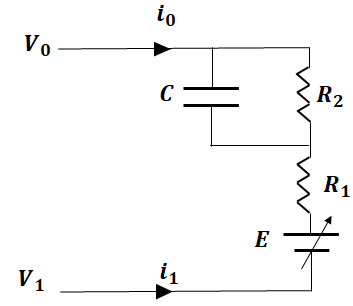2.3 Temperature Effects in battery

When a cell is charged or discharged, the temperature of the cell varies, and accordingly, ion diffusion in the solid is affected. The temperature of a cell as an energy equation under isothermal conditions is :

(11)
$\rho C_{p}\frac{\partial T}{\partial t}=\left(Q_{gen}-q\right)$

where

$\rho$ : density of the cell

$C_{p}$ : heat capacity of the cell

$Q_{gen}$ : heat generation rate per unit volume.

Heat flux between a cell and the surroundings is expressed as:

(12)
$q=~ \frac{h}{d}\left(T-T_{a}\right)$

where

$h$ : heat transfer coefficient

$d$ : thickness of cell

$T_{a}$ : ambient temperature

A battery will generate heat during charge or discharge operations. These operations will be calculated based on a voltage difference such as the one between the OCV (open circuit voltage) and terminal voltage. In general, heat generation is expressed as a sum of reversible and irreversible heat generation terms. The irreversible heat source term is the difference between the voltage and OCV. The reversible heat source term is the change of entropy of OCV over a given temperature.

(13)
$~ Q_{gen}=\frac{1}{V}~ \left(U_{OCV}-V_{T}\right)-\frac{1}{V}~ \left(T\cdot \frac{\partial U_{OCV}}{\partial T}\right)~$

where

$U_{OCV}$: open circuit voltage

$V_{T}$: terminal voltage

$T$ : temperature of the battery

For a small change in the battery, the temperature increases, and the effects will be observed in future use.

Table 1. Characteristics of Li-Polymer Battery.
 Parameters Li polymer Current [A] 16 Rated Voltage [V] 3.7 Voltage [V] 12.0 Power [W] 177 Circuit 3S1P

3. kNN Algorithm Design for SOC and DOD Model Training

Machine learning methods are used to predict a model, but one must be selected based on our requirement and error percentage deviation. In this study, the kNN regression algorithm was used to predict the values of SOC of a battery based on the voltage degradation parameter. In this method, the target is predicted based on the Euclidean distance method. The Euclidean distance method is applied to test the nearest neighbor point.

After calculating nearest neighbors, the weighted average of nearest neighbors is used as a final prediction. K layers must be chosen based on the accurate comparison of trained and tested data. We chose the K nearest neighbors with the calculated distance method. Among the obtained K neighbors, we count the number of data points and categories of the maximum number of neighbors. Finally, a predicted value is obtained as output.

This study was carried out with a lithium-polymer pouch battery with a nominal voltage of 3.7 V and rated current of 16 A. The pack is made by a combination of 3 cells arranged in series (3S1P) with a maximum power of 177 W.

Then battery SOC is calculated as given in Eq. (14) :

(14)
$SOC_{\textit{Estimation}}=\frac{C_{\textit{capacity}}}{C_{\textit{rated}}}\times 100%$

where C$_{\mathrm{capacity}}$ is the initial capacity of the battery, and C$_{\mathrm{rated}}$ is the capacity of the battery currently available. In this study, the equationwas used to calculate the time for SOC estimation and the SOC origin of the lithium battery pack. This calculated SOC is sent to the kNN classifier for the prediction of SOC. Capacity'' is the initial capacity of the battery, and Crated'' is the capacity of the battery currently available.

This calculated SOC is sent to the kNN classifier for the prediction of SOC. The DOD is also an important parameter to estimate the life of a battery. High SOC and low DOD will decrease the battery life rapidly. DOD is expressed as in this equation:

(15)
$DOD=1-\frac{C_{\textit{capacity}}}{C_{\textit{rated}}}$

The battery life cycle will be decreased over time due to many factors and interconnected degradation mechanisms. Hence, operation cycle conditions play a vital role in identifying parameters. Hence, the number of cycles is considered as a common parameter, and a C-rate of 0.5C and ambient temperature (25$^{\circ}$C) are the most important variables .

The experiment results were sent to the machine learning algorithm and trained to obtain the result of SOC. We then compared this predicted result with an experiment result.

The predicted output accuracy has to be calculated by the error calculation in between the predicted and actual SOC values. It is given as the mean absolute error (MAE), which is defined as the difference of the actual value and the predicted value :

(16)
$MAE=\frac{1}{n}\sum _{i=1}^{n}\left(T_{i}-A_{i}\right)$

where n is the number of samples, T$_{\mathrm{i}}$ is the predicted value, and A$_{\mathrm{i}}$ is the actual value. In this study, the equation was used to calculate the time for SOC estimation and the SOC origin of the lithium battery pack.

The distance to be calculated is given by the Euclidean distance in Eq. (17):

(17)
$Y=\sqrt{\sum _{i=1}^{N}w_{n}\left(x_{t}-x_{i}\right)^{2}}$

where N is the number of parameters that are dependent, x$_{\mathrm{t}}$ is the parameter of a test point\textit{, x}$_{i}$ is the parameter of a training point, and w$_{\mathrm{n}}$ is the weight of the parameter and is given as Eq. (18):

(18)
$w_{n}~ =\frac{~ ~ ~ ~ \left(\frac{1}{y_{t,i}}\right)^{t}}{\sum _{i=1}^{k}\left(\frac{1}{y_{t,i}}\right)^{t}}$

where y$_{\mathrm{t,i}}$ is the distance between the nearest test and neighbor points.

Sample calculation of Euclidean distance is explained as follows based on experiment results. If we substitute the theoretical SOC in Eqs. (17) and (18) (x$_{\mathrm{t}}$ = 88, x$_{\mathrm{i}}$ = 89, which are the SOC with respect to time), from these coordinates, we can easily calculate the distance between nearest and neighboring points. We obtained y$_{\mathrm{t,i}}$= 1.280 and w$_{\mathrm{n}}$ = 0.8194, and the Euclidean distance calculated by this method was obtained as y = 1.31 mm. In this way, we can calculate the shortest distance for each value based on the kNN layer number to predict SOC, which is more accurate with the experimental SOC.

Fig. 5. Network Structure for Training Model.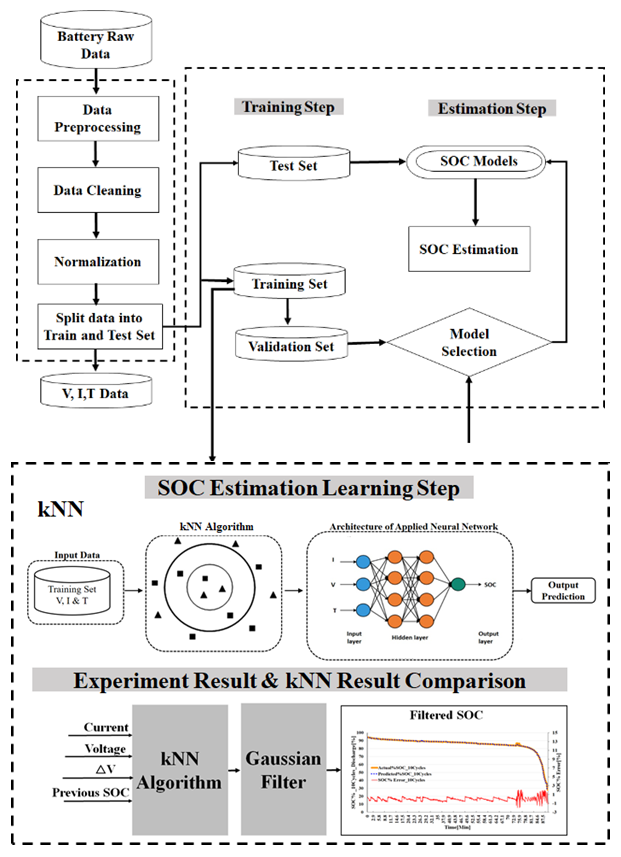4. Experimental Results

The experiment setup consists of charger and discharger units. The battery is subjected to a constant current discharge method with a discharge rate of 0.5C. In the procedure of the experiment, initially, the battery charges to 3.9 V, rests for one hour, and then discharges to 2.7 V with constant current. The experiment process is explained in the steps below:

[Step 1] The lithium battery pack consists of 3 cells which are fully charged with constant current of 8 A, and this state is called 100% SOC.

[Step 2] After completion of charging, the entire battery pack.

[Step 3] The lithium battery pack is discharged to the cutoff voltage.

[Step 4] Voltage and current are measured during discharge and sent to a computer with sensors.

[Step 5] Procedure steps (1) to (4) are repeated for every cycle, and data is collected during the process. If temperatures are increasing rapidly, we note corresponding temperatures also.

[Step 6] The recorded data that was measured is sent to the kNN model for the training process.

[Step 7] The data is learned using the kNN model.

[Step 8] The actual SOC estimation result and the predicted or learned SOC estimation result are compared.

[Step 9] The mean absolute error is used to calculate the error rate.

The experiment setup has been realized as shown in Fig. 7. A discharge unit, power supply, battery, and computer with a current sensor and voltage sensors were used for this experiment. After charging a battery up to the required maximum rated voltage, it rests for 30 to 60 minutes and then starts to discharge. We then collect the charge and discharge voltage and current with respect to time, and it is stored in a computer. The values of voltage in 10 cycles were the same. Hence, the averages for every 10, 50, 100, and 150 cycles were collected. We calculated the theoretical SOC of the battery and compared it with the proposed kNN algorithm.

The result of the experiment and predicted SOC with machine learning model was obtained. The deviation between the measured and predicted SOC was evaluated from the MAE method. The details of the measured and predicted result with error between these models are explained in the next section.

Fig. 8. Comparison of SOC with Experiment and kNN Model In Battery During Discharge: (a) Experiment Result, (b) 10 Cycles, (C) 50 Cycles, (D) 100 Cycles and (E) 150 Cycles.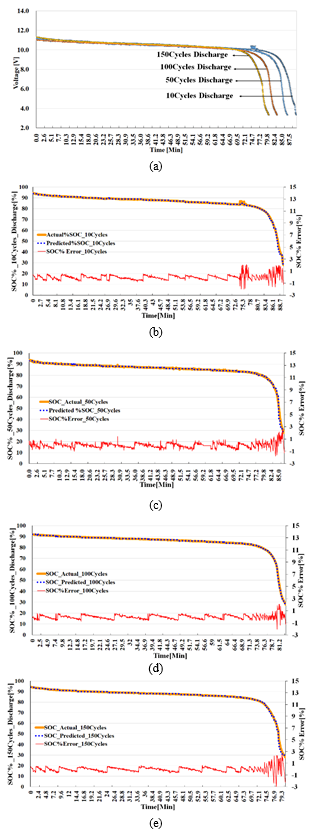4.1 Result of Voltage and SOC in Li-polymer Battery during Discharge

The experimental results shown below in Fig. 8 are graphs that compare between the theoretical SOC estimation and predicted SOC (Figs. 8(b) to (e)). The MAE has been calculated and shows a deviation of 0.74[%] with the original calculated SOC value, which was the lowest value. The yellow line indicates experiment data, and the blue dotted line shows the kNN method of charge prediction. The results are satisfactory.

From the experimental result, we observed that the voltage is gradually degraded when the battery underwent consecutive cycles of discharge. For 10 cycles, the constant voltage is reduced, and complete discharge of battery takes 90 minutes. But after 50 cycles, a rapid decrease in the voltage is observed, and within 80 minutes, the battery discharged completely. This result is compared with the predicted SOC with the kNN. The predicted and experimental results have an MAE of 0.74[%]. Hence, the predicted SOC is very near the measured or calculated SOC value. The MAEs with the kNN and experiment results were calculated, and we obtained a lowest value of 0.74[%].

MAE graphs are plotted for 10, 50, 100, and 150 cycles in Figs. 8(a) to (d). They show the error value representation on the y axis at the corresponding time on the x axis. Similarly, for discharge, the MAE is range between below 0.5 deviation. The experiment and the MAE are satisfactory.

4.2 Result of Voltage and SOC in Li-polymer Battery during Charging

The same experiment was repeated for charge in a constant current method process with a charge rate of 0.5C. We observed that the voltage is gradually increased when

the battery undergoes consecutive cycles of charge. For 10 cycles, the constant voltage is increased to 4.0 V from 2.7 V, and complete charge of the battery takes 90 minutes. But after 50 cycles, a rapid increase in the voltage was observed, and within 80 minutes, the battery charged completely.

This result was compared with the predicted SOC with the kNN machine learning. The predicted and the experimental results have an MAE of 0.74[%]. Hence, the predicted SOC is very near the measured or calculated SOC value. The results from the experiment are indicated in Fig. 9(a), and the comparison of predicted and experimental results with the MAE is presented in the graphs in Figs. 9(b) to (e).

Tables 2 and 3 show a comparison of temperatures at the ends of cycles during charge and discharge of the battery based on the operating time and voltage. It is observed that with increase in the operation time, the battery temperature also gradually increases.

Table 2. Charging Experiment Results.
 Charging Time [Min] Voltage [V] Temperature [°C] 10 Cycles 89.3 12.2 28.0 50 Cycles 85.6 12.2 29.2 100 Cycles 82.8 12.2 31.1 150 Cycles 79.6 12.2 32.3
Table 3. Discharge Experiment Results.
 Discharge Time [Min] Voltage [V] Temperature [°C] 10 Cycles 88.7 3.2 28.2 50 Cycles 85.0 3.2 30.3 100 Cycles 81.2 3.2 33.1 150 Cycles 79.3 3.2 37.2
Fig. 9. Comparison of SOC with Experiment and kNN Model in Battery during Charge: (a) Experiment Result, (b) 10 cycles, (c) 50 Cycles, (d )100 Cycles, (e) 150 Cycles.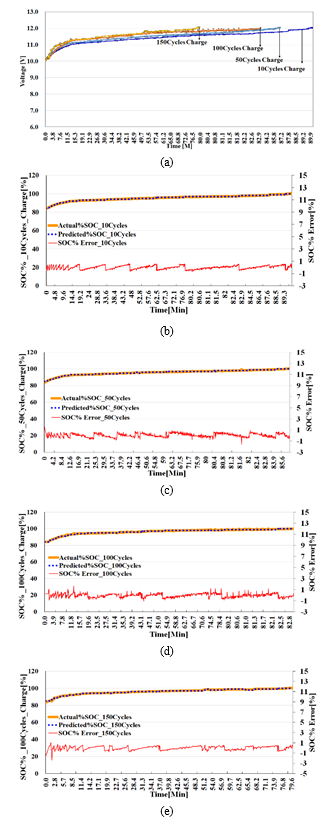5. Conclusion

In this paper, we proposed an SOC estimation method for a battery using the kNN machine learning method. The following conclusions were made in this study:

$\color{color-4}{·}$ Experiments were conducted at room temperatures. The proposed method was used to estimate SOC in real time.

$\color{color-4}{·}$ A lithium-polymer battery with a nominal capacity of 4 V and 16 A was used in this study.

$\color{color-4}{·}$ The battery was charged and discharged with the constant current method at a rate of 0.5C.

$\color{color-4}{·}$ During the experiment, voltage variations in the battery after 10, 50, 100, and 150 cycles were observed.

$\color{color-4}{·}$ At up to 10 cycles, the battery performance was good and gradually decreased after 150 cycles.

$\color{color-4}{·}$ This experiment result was compared with the kNN machine learning method for instant prediction of SOC

$\color{color-4}{·}$ Two datasets for prediction were used: one for voltage degradation during charge at different cycles and one for voltage during charging.

$\color{color-4}{·}$ After training and prediction, we evaluated the results and compared them with the experiment and kNN method.

$\color{color-4}{·}$ The kNN algorithm had an MAE of 0.74[%] and achieved 98% accuracy for the two different types of batteries. This model has an advantage of overcoming complex solvers numerically and analytically.

In the future, work will be carried out with different machine learning algorithms by observing temperatures changes in the battery with a linear regression algorithm.

ACKNOWLEDGMENTS

This work was supported by the Korea Ministry of Trade, Industry and Energy under the grant of The development of high strength lightweight aluminum battery package and PCM-BTMS for high safety and battery efficiency improvement of electrical vehicle 2021.''

REFERENCES

1
Zhang Wenqiang, et al. , 2018, A durable and safe solid-state lithium battery with a hybrid electrolyte membrane., Nano Energy, Vol. 45, pp. 413-4192
Song Xiangbao, et al. , 2019, Combined CNN-LSTM network for state-of-charge estimation of lithium-ion batteries., IEEE Access, Vol. 7, pp. 88894-889023
Chen C., Xiong R., Yang R., Shen W., Sun F., 2019, State-of-charge estimation of lithium-ion battery using an improved neural network model and extended Kalman filter., J. Clean. Prod., Vol. 234, pp. 1153-11644
Huang Deyang, et al. , 2019, A model-based state-of-charge estimation method for series-connected lithium-ion battery pack considering fast-varying cell temperature., Energy, Vol. 185, pp. 847-8615
Abbas G., Nawaz M., Kamran F., 2019 January, Performance comparison of NARX & RNN-LSTM neural networks for LiFePO4 battery state of charge estimation., In 2019 16th International Bhurban Conference on Applied Sciences and Technology (IBCAST) IEEE., pp. 463-4686
Lu Languang, et al. , 2013, A review on the key issues for lithium-ion battery management in electric vehicles., Journal of power sources, Vol. 226, pp. 272-2887
Meng Jinhao, et al. , 2017, An overview and comparison of online implementable SOC estimation methods for lithium-ion battery., IEEE Transactions on Industry Applications, Vol. 54, No. 2, pp. 1583-15918
Kim Jonghoon, et al. , 2011, Stable configuration of a Li-ion series battery pack based on a screening process for improved voltage/SOC balancing., IEEE Transactions on Power Electronics, Vol. 27, No. 1, pp. 411-4249
Lee P. Y., et al. , 2017, Screening method using the cells deviation for Li-ion battery pack of the high power application., Proceedings of the KIPE Conference. The Korean Institute of Power Electronics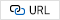10
He Wei, et al. , 2018, A physics-based electrochemical model for lithium-ion battery state-of-charge estimation solved by an optimised projection-based method and moving-window filtering., Energies, Vol. 11, No. 8, pp. 212011
You Hyun Woo, et al. , 2018, Analysis of equivalent circuit models in lithium-ion batteries., AIP Advances, Vol. 8, No. 12, pp. 12510112
Birkl B., Christoph R., et al. , 2017, Degradation diagnostics for lithium ion cells., Journal of Power Sources, Vol. 341, pp. 373-38613
Li Xueyan , Meng Xiao , Song-Yul Choe. , 2013, Reduced order model (ROM) of a pouch type lithium polymer battery based on electrochemical thermal principles for real time applications., Electrochimica Acta, Vol. 97, pp. 66-7814
Gao, Lijun , Shengyi Liu , Roger A. Dougal. , 2002, Dynamic lithium-ion battery model for system simulation., IEEE transactions on components and packaging technologies, Vol. 25, No. 3, pp. 495-50515
Chang Wen-Yeau., 2013, The state of charge estimating methods for battery: A review., International Scholarly Research Notices 201316
Sidhu, Manjot S. , Deepak Ronanki , Sheldon Williamson. , 2019, Hybrid state of charge estimation approach for lithium-ion batteries using k-nearest neighbour and gaussian filter-based error cancellation., 2019 IEEE 28th International Symposium on Industrial Electronics (ISIE). IEEEAuthor

Teressa Talluri is a Ph.D. student in Busan University of Foreign Studies. She is a professor in the Mechanical Engineering Department at KL University India. She also received the best faculty award. She received a master’s of technology degree from Jawaharlal Nehru Technological University, Kakinada, India. She was the university topper in her masters course. She received a bachelor’s degree from Jawaharlal Nehru Technological University, Hyderabad, India. She also researched about battery thermal management with a new medium called phase-change materials and observed a good result in that research. Her areas of interest in research are thermal management in an electric vehicle battery, heat transfer, artificial intelligence, and robotics.

Hee Tae Chung has been a professor in the Department of Electronic and Robot Engineering in Busan University of Foreign Studies, Busan, Korea, since 1997. He received his M.S. and Ph.D. degrees in electronic engineering from Kyungpook National University, Dague, Korea, in 1988 and 1996, respectively. Between 1996 and 1997, he worked as a Patent Examiner in the Korean Industrial Property Office. His current research areas include the application of intelligent control to robot systems, adaptive control, and deep learning with neural networks.

Kyoo Jae Shin is a professor of Intelligence Robot Science at Busan University of Foreign Studies (BUFS), Busan, South Korea. He is the director of the Future Creative Science Research Institute at BUFS. He received his B.S. degree in electronics engineering in 1985 and an M.S. degree in electrical engineering from Cheonbuk National University (CNU) in 1988, and he received his Ph.D. degree in the electrical science from Pusan National University (PNU) in 2009. Dr. Shin was a professor of the Navy Technical Education School and a main director for research associates of a dynamic stabilization system in Dusan Defense Weapon Research Institute. Also, he has researched and developed a fish robot, submarine robot, automatic bug spray robot in a glass room, automatic milking robot using a manipulator, personal electrical vehicle, smart accumulated aquarium using a heat pump, solar tracking system, 3D hologram system, and gun/turret stabilization system. He is interested in intelligent robots, image signal processing application systems, and smart farms and aquariums using new energy.